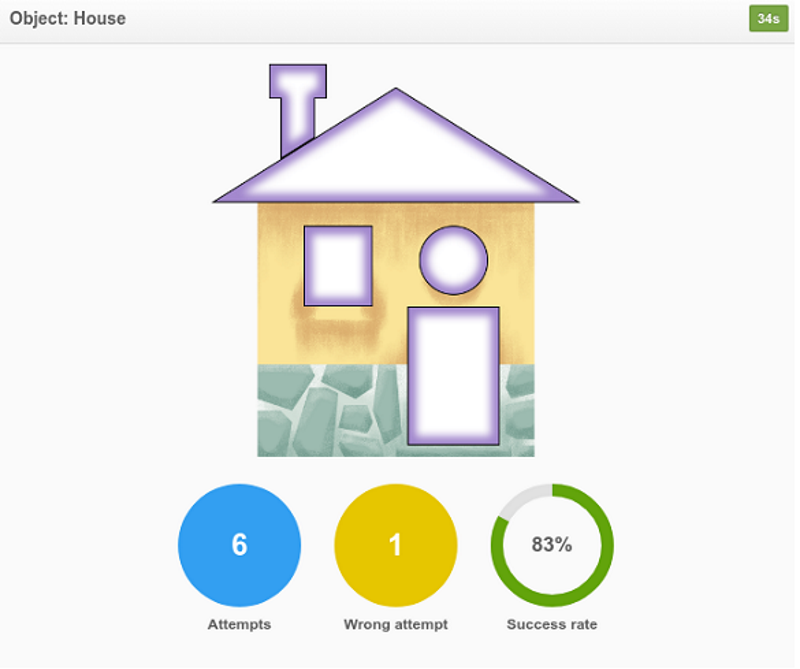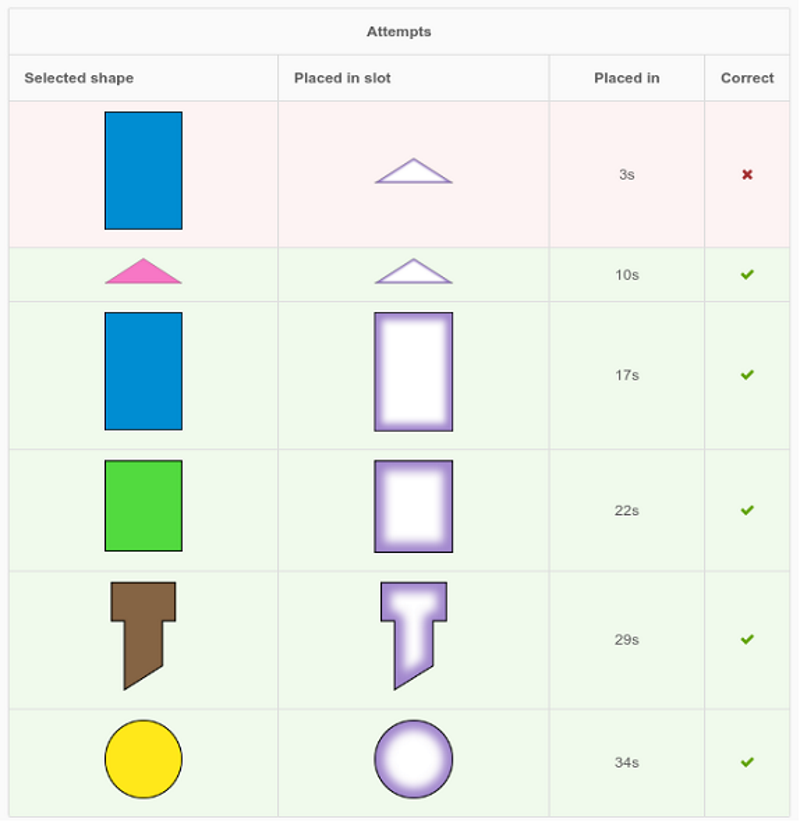### Shape in Place

In the “Shape in Place” game, a student composes simple 2D shapes to form larger shapes and real-world objects. They must drag a shape and drop it at the appropriate slot. This colored picture puzzle helps children expand their vocabulary, recognition of shapes and reinforces their ability to categorize and to place different shapes for creating real-world image representations of a boat, a house, a van, a sky-rocket, etc. This strengthens problem solving, concentration, and gross motor skills such as grabbing, middle-line crossing and hand stability. When students attempt to position a shape in its place, it will only fit if it is properly put in the right space.

The game can be made even easier by activating the “help option” meaning that the puzzle piece and the related position, where the piece goes, will be highlighted for few seconds thus guiding the student.A shape can be picked and dragged by a student either with grab & move interaction style or with time-delay selection and movement.  The teacher/therapist can choose the interaction style that is compatible with a student's motor skills level.

The teacher/therapist can adjust the timer for the completion of the given composing task. Also, students are asked to complete various images depending on their interests such that they learn to place different objects into different containers.

Are you ready to create brightly coloured pictures?

## Supported goals in Common CoreCaliforniaFloridaNevadaNew YorkNorth CarolinaPennsylvaniaSouth CarolinaTenesseeTexasVirginia

CCSS.MATH.CONTENT.PK.G.2b
Math
Create and build shapes from components (e.g., sticks and clay balls).
CCSS.MATH.CONTENT.K.G.B.5
Math
Model shapes in the world by building shapes from components (e.g., sticks and clay balls) and drawing shapes.
CCSS.MATH.CONTENT.K.G.B.6
Math
Compose simple shapes to form larger shapes. For example, "Can you join these two triangles with full sides touching to make a rectangle?"
CCSS.MATH.CONTENT.1.G.A.2
Math
Compose two-dimensional shapes (rectangles, squares, trapezoids, triangles, half-circles, and quarter-circles) or three-dimensional shapes (cubes, right rectangular prisms, right circular cones, and right circular cylinders) to create a composite shape, and compose new shapes from the composite shape.
CA.MATH.1.G.2
Math
Reason with shapes and their attributes. Compose two-dimensional shapes (rectangles, squares, trapezoids, triangles, half-circles, and quarter-circles) or three-dimensional shapes (cubes, right rectangular prisms, right circular cones, and right circular cylinders) to create a composite shape, and compose new shapes from the composite shape. (Students do not need to learn formal names such as “right rectangular prism.”)
CA.MATH.K.G.5
Math
Analyze, compare, create, and compose shapes. Model shapes in the world by building shapes from components (e.g., sticks and clay balls) and drawing shapes.
CA.MATH.K.G.6
Math
Analyze, compare, create, and compose shapes. Compose simple shapes to form larger shapes. For example, "can you join these two triangles with full sides touching to make a rectangle?”
MAFS.K.G.2.AP.6a
Math
Compose a larger shape from smaller shapes
OEL.FL.MATH.PK.G.2b
Math
Create and build shapes from components (e.g., sticks and clay balls).
MAFS.1.G.1.AP.2a
Math
Draw or build two- and three-dimensional shapes
MAFS.K.G.2.5
Math
Model shapes in the world by building shapes from components (e.g., sticks and clay balls) and drawing shapes.
NVAC.MATH.K.G.B.6
Math
Analyze, compare, create, and compose shapes. Compose simple shapes to form larger shapes. For example, "can you join these two triangles with full sides touching to make a rectangle?”
NVAC.MATH.K.G.B.5
Math
Analyze, compare, create, and compose shapes. Model shapes in the world by building shapes from components (e.g., sticks and clay balls) and drawing shapes.
NVAC.MATH.1.G.A.2
Math
Reason with shapes and their attributes. Compose two-dimensional shapes (rectangles, squares, trapezoids, triangles, half-circles, and quarter-circles) or three-dimensional shapes (cubes, right rectangular prisms, right circular cones, and right circular cylinders) to create a composite shape, and compose new shapes from the composite shape. (Students do not need to learn formal names such as “right rectangular prism.”)
NY.MATH.PK.G.2b
Math
Create and build shapes from components (e.g., sticks and clay balls).
NY.MATH.K.G.5
Math
Model shapes in the world by building shapes from components (e.g., sticks and clay balls) and drawing shapes.
NY.MATH.K.G.6
Math
Compose simple shapes to form larger shapes. For example, “Can you join these two triangles with full sides touching to make a rectangle?”
NY.MATH.1.G.2
Math
Compose two-dimensional shapes (rectangles, squares, trapezoids, triangles, half-circles, and quarter-circles) or three-dimensional shapes (cubes, right rectangular prisms, right circular cones, and right circular cylinders) to create a composite shape, and compose new shapes from the composite shape.4
NC.MATH.K.G.5
Math
Model shapes in the world by: 1. Building and drawing triangles, rectangles, squares, hexagons, circles. 2. Building cubes, cones, spheres, and cylinders.
NC.MATH.1.G.2
Math
Create composite shapes by: 1. Making a two-dimensional composite shape using rectangles, squares, trapezoids, triangles, and half-circles naming the components of the new shape. 2. Making a three-dimensional composite shape using cubes, rectangular prisms, cones, and cylinders, naming the components of the new shape.
NC.MATH.K.G.6
Math
Compose larger shapes from simple shapes.
PA.CC.MATH.K.G.B.6
Math
Compose simple shapes to form larger shapes. For example, "Can you join these two triangles with full sides touching to make a rectangle?"
PA.CC.MATH.1.G.A.2
Math
Compose two-dimensional shapes (rectangles, squares, trapezoids, triangles, half-circles, and quarter-circles) or three-dimensional shapes (cubes, right rectangular prisms, right circular cones, and right circular cylinders) to create a composite shape, and compose new shapes from the composite shape.
PA.CC.MATH.PK.G.2b
Math
Create and build shapes from components (e.g., sticks and clay balls).
PA.CC.MATH.K.G.B.5
Math
Model shapes in the world by building shapes from components (e.g., sticks and clay balls) and drawing shapes.
SC.MATH.1.G.2
Math
Combine two-dimensional shapes (i.e., square, rectangle, triangle, hexagon, rhombus, and trapezoid) or three-dimensional shapes (i.e., cube, rectangular prism, cone, and cylinder) in more than one way to form a composite shape.
SC.MATH.K.G.5
Math
Draw two-dimensional shapes (i.e., square, rectangle, triangle, hexagon, and circle) and create models of three-dimensional shapes (i.e., cone, cube, cylinder, and sphere).
SC.MATH.PK.G.2b
Math
Create and build shapes from components (e.g., sticks and clay balls).
SC.MATH.K.G.2
Math
Identify and describe a given shape and shapes of objects in everyday situations to include two-dimensional shapes (i.e., triangle, square, rectangle, hexagon, and circle) and three-dimensional shapes (i.e., cone, cube, cylinder, and sphere).
No supported goals.
TEKS.MATH.1.6.F
Math
Compose two-dimensional shapes by joining two, three, or four figures to produce a target shape in more than one way if possible;
TEKS.MATH.K.6.F
Math
Create two-dimensional shapes using a variety of materials and drawings.
TEKS.MATH.PK.G.2b
Math
Create and build shapes from components (e.g., sticks and clay balls).
VASOL.MATH.1.G.13
Math
The student will construct, model, and describe objects in the environment as geometric shapes (triangle, rectangle, square, and circle) and explain the reasonableness of each choice.
VASOL.MATH.5.G.13a
Math
The student, using plane figures (square, rectangle, triangle, parallelogram, rhombus, and trapezoid), will develop definitions of these plane figures

## Settings

### I CAN statement

Music

Disable/enable background music.

• On
• Off
Sound effects

Disable/enable sound effects while selecting an item.

• On
• Off

I CAN understand that there are sounds when I choose an item.

Select hand
• Left
• Right

I CAN select the “Play” button with my left hand.

Time to select item

Time to remain stable to select an item.

Reduce the time if the child shows stability.

• 0.5 sec
• 1 sec
• 1.5 sec
• 2 secs
• 2.5 secs
• 3 secs

I CAN keep my hand stable in an upright position for 3 seconds to pick a shape.

Interaction mode

A shape can be picked and dragged either by the child with grab & move interaction style or time-delay selection and move. The teacher/therapist can choose the interaction style that is compatible with a child's motor skills.

• Grab and move
• Delay and move

I CAN hold my hand stable on top of a shape in order to pick it and drag it to the right slot. Also, I CAN grab the shape and move it to the slot.

Timer

Time available to complete an image by placing all the shapes into the right slots.

Disable this if a student feels pressure.

• On
• Off

I CAN complete an image within 1 minute.

Timer value
• 1 min
• 1.5 min
• 2 mins
• 2.5 mins
• 3 mins
• 3.5 mins
• 4 mins
• 4.5 mins
• 5 mins
Game lives

Enable or disable game lives.

Game lives are the three little hearts in the top right corner of the screen.

• On
• Off

I CAN notice that a heart disappears each time I am wrong.

I CAN play the game with game lives.

Content language
• English
• Spanish
• Greek
Help

It is enabled after a certain period of idle time or after three wrong attempts in a row. The puzzle piece and the related hole, where the piece goes will be highlighted for few seconds, thus guiding the child.

• On
• Off

I CAN get helped in case I cannot identify a right match for shape and slot.

Game mode

Children are asked to complete various objects depending on their interests such that they learn to place different shapes into different slots.

• Model shapes in the real world
• Compose simple shapes to form larger shapes
• Create a composite shape

I CAN place different shapes into the correct slots and create a dinosaur.

Objects
• House
• Train
• Space-Rocket
• Ship
• Van
• Hexagon 1
• Trapezoid
• Square 1
• Square 2
• Square 3
• Hexagon 2
• Hexagon puzzle
• Square puzzle 1
• Square puzzle 2
• Square puzzle 3
• Square puzzle 4
• Building
• Construction
• Castle
• Car
• Dinosaur

## ReportsPie chart data

This shows statistics on the number of objects the child completed correctly, the number of incomplete objects and the total number of correct and wrong attempts. Also, we can see the total time spent playing.This shows the amount of time that the student spent on each object and presents the object’s original screen.The educator/teacher can see in detail the student’s choices for each specific shape which might reveal possible misconceptions and certain patterns of cases, where the student faces difficulties.